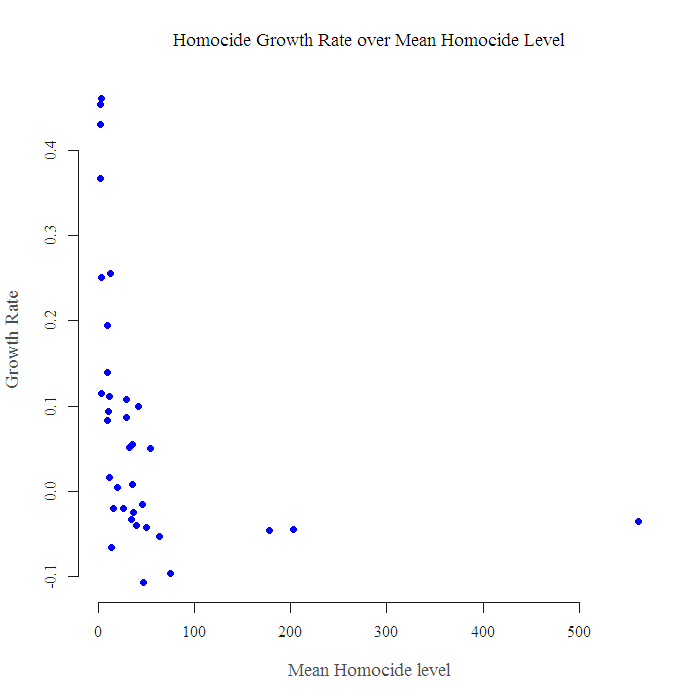[This article was first published on Eran Raviv » R, and kindly contributed to R-bloggers]. (You can report issue about the content on this page here)
Want to share your content on R-bloggers? click here if you have a blog, or here if you don't.

When I was searching for data about U.S prison population, for another post, I run across eurostat, a nice source for data to play around with. I pooled some numbers, specifically homicides recorded by the police. A panel data for 36 cities over time, from 2000 to 2009. Lets see which are the cities that have problems in this area.

The first few lines look like:

  CITIES.TIME 2000 2001 2002 2003 2004 2005 2006 2007 2008 2009
1   Amsterdam   44   33   24   39   27   32   17   32   17   33
2      Athina   52   47   46   47   41   49   43   68   69   70
3     Belfast   21   15   16    4    8   15    9    6    2    6
4     Beograd   93   70   42   40   42   49   52   51   38   27

The graph for the growth rate $$(\frac{x_t}{x_{t-1}} – 1)$$ looks like this:

Despite it being very colorful, its not really useful. We can see many spikes meaning an increase of 300%, which of course derived from a city with a jump in homicide from one to three cases that year. Hadley Wickham gave a nice NBA example, where you better not pick the player with best percent accuracy, you will just end up with 5 people that shoot 100% which is based on one attempt at the hoop. Now what? We can use the level of homicide as a measure for the size of the city, so we get the following figure:Now we look for points (cities) that sit in the upper right quadrant. Which will mean, high growth rate coupled with high level of homicide. A way to do that is to order the observation according to the strange expression: $$\frac{Homicide Rate}{\frac{1}{average level} }$$

When Average level is low, the expression is low, and the reverse. the nominator is clear I hope. Now we order them and bar-plot the most problematic cities:

That is it. Thanks for reading. Code and references are below.

1. Of course, I could have gotten some data on the actual population in these cities, but where is the fun in that?
2. Some of the most dangerous cities are not in, e.g. Marseilles-France or Sofia-Bulgaria, they are just not in the dataset.
Code:

?View Code RSPLUS
 t2 = read.table("/homocide1.txt", sep = "\t", header = T) head(t2, 4) ; dim(t2) ; names(t2) names(t2)[2:11] = seq(2000,2009,1) # drop the time index matplot(t(t2[,2:NCOL(t2)]), ty = "b", pch = 1) t22 = t2[,2:11]+1 # Avoid inf in the rate of change rt2 = t22[,2:NCOL(t22)]/t22[,1:(NCOL(t22)-1)] - 1 matplot(t(rt2), ty = "b", pch = 1, xaxt = "n", xlab = "Time", ylab = "Growth Rate", cex.lab = 1.5, main = "Growth Rate over Time") axis(side = 1, at = c(1:9), labels = seq(2001,2009,1)) plot(apply(rt2,1,mean)~apply(t22[,2:10],1,mean), pch = 19, xlab = "Mean Homocide level", cex.lab = 1.2, ylab = "Growth Rate", col = "blue", main = "Homocide Growth Rate over Mean Homocide Level") a1 = apply(rt2,1,mean)/(1/apply(t22[,2:10],1,mean)) # the funny expression x11() d = 1.5 # just few largest a2 = sort(a1[a1>d]) barplot(a2, names.arg = t2[c(as.numeric(names(a2))),1], horiz = F, cex.names = 1, space = 0.03, angle = 45, density=NULL, col = "lightblue",width = .2, main ="Most Dengerous Cities" )

Related:

To leave a comment for the author, please follow the link and comment on their blog: Eran Raviv » R.

R-bloggers.com offers daily e-mail updates about R news and tutorials about learning R and many other topics. Click here if you're looking to post or find an R/data-science job.
Want to share your content on R-bloggers? click here if you have a blog, or here if you don't.

# Never miss an update! Subscribe to R-bloggers to receive e-mails with the latest R posts.(You will not see this message again.)

Click here to close (This popup will not appear again)